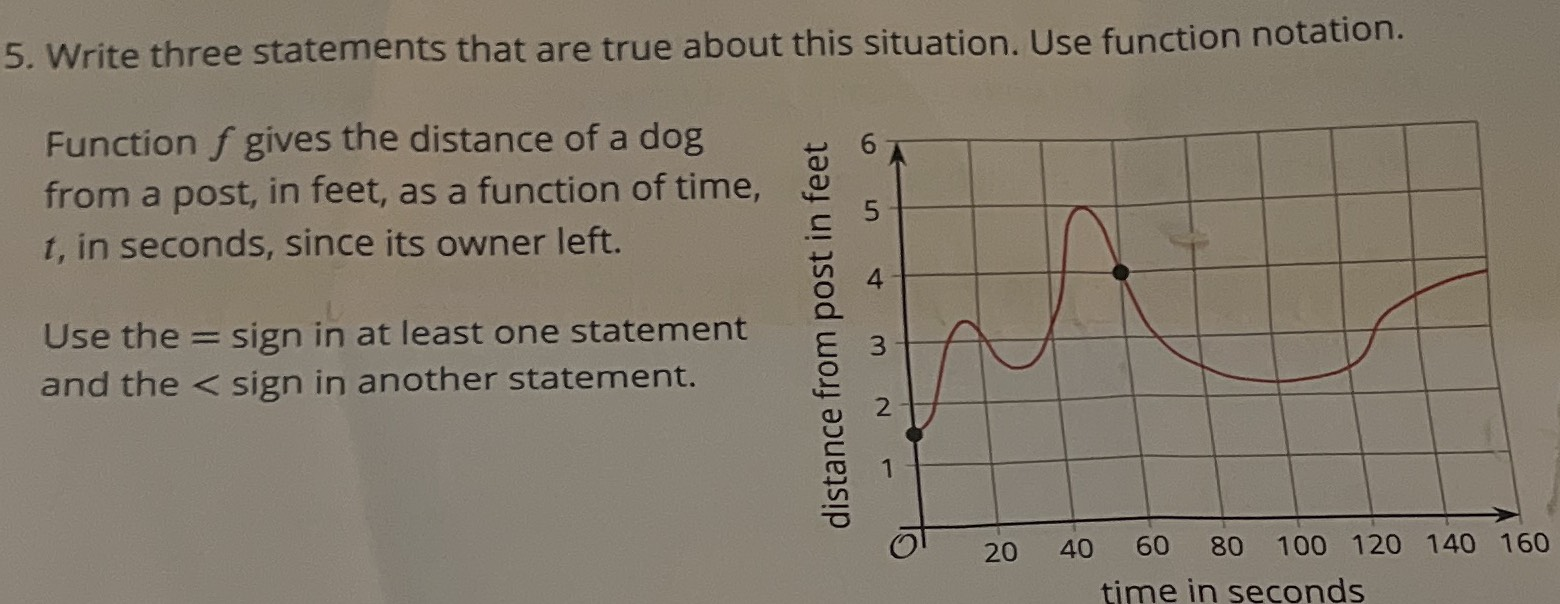### ¿Todavía tienes preguntas de matemáticas?

Pregunte a nuestros tutores expertos
Algebra
PreguntaFunction $$f$$gives the distance of a dog from a post, in feet, as a function of time, $$t$$, in seconds, since its owner left. Use the $$=$$sign in at least one statement and the $$<$$sign in another statement.